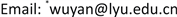﻿ 两类周期点的局部动力学性态 Local Dynamical Behavior of Two Classes of Periodic Points

Vol. 08  No. 01 ( 2019 ), Article ID: 28387 , 5 pages
10.12677/AAM.2019.81003

Local Dynamical Behavior of Two Classes of Periodic Points

Xiaolin Li, Yan Wu*

School of Mathematics and Statistics, Linyi University, Linyi ShandongReceived: Dec. 11th, 2018; accepted: Jan. 2nd, 2019; published: Jan. 9th, 2019ABSTRACT

In this paper, by studying the existence of the conformal isomorphism of rational function near its attracting periodic point and superattracting periodic point, we give the local dynamical behavior of rational function at these two kinds of periodic points.

Keywords:Rational Function, Attracting Periodic Point, Superattracting Periodic Point1. 引言

2. 预备知识

${z}_{0}={f}^{0}\left({z}_{0}\right),{z}_{1}={f}^{1}\left({z}_{0}\right),{z}_{2}={f}^{2}\left({z}_{0}\right),\cdots ,{z}_{n}={f}^{n}\left({z}_{0}\right),\cdots$

$\left\{{z}_{0},{z}_{1}=f\left({z}_{0}\right),{z}_{2}={f}^{2}\left({z}_{0}\right),\cdots ,{z}_{p-1}={f}^{p-1}\left({z}_{0}\right)\right\}$

1) 如果 $|\lambda |<1$ ，称 ${z}_{0}$ 为吸性周期点，( $\lambda =0$ 时称为超吸性不周期点)；

2) 如果 $|\lambda |>1$ ，称 ${z}_{0}$ 为斥性周期点；

3) 如果 $|\lambda |=1$ 且对某个整数n成立 ${\lambda }^{n}=1$ ，称 ${z}_{0}$ 为有理中性周期点；如果 $|\lambda |=1$ 但是 ${\lambda }^{n}\ne 1$ ，称 ${z}_{0}$ 为无理中性周期点。

$\phi \left(f\left(z\right)\right)=g\left(\phi \left(z\right)\right)$ .

3. 周期点附近的动力学性态

$f\left(z\right)=\lambda z+{a}_{2}{z}^{2}+{a}_{3}{z}^{3}+\cdots$ . (3.1)

$\phi \circ f\left(z\right)=\lambda \phi \left(z\right)$

$|{f}^{n}\left(z\right)|<{c}^{n}|z|<\delta {c}^{n}$ .

$|f\left(z\right)-\lambda z| ,

$z\in {\text{Δ}}_{\delta }$ 时，

$|{f}^{n+1}\left(z\right)-\lambda {f}^{n}\left(z\right)| .

$|{\phi }_{n+1}\left(z\right)-{\phi }_{n}\left(z\right)|=\frac{1}{{|\lambda |}^{n+1}}|{f}^{n+1}\left(z\right)-\lambda {f}^{n}\left(z\right)|<\frac{K{\delta }^{2}}{|\lambda |}{\left(\frac{{c}^{2}}{|\lambda |}\right)}^{n}$ .

$\frac{{c}^{2}}{|\lambda |}<1$ 知， ${\phi }_{n}$${\Delta }_{\delta }$ 内是一致收敛的Cauchy序列。记 $\phi$${\phi }_{n}$ 的极限函数，取 $U={\phi }^{-1}\left({\Delta }_{r}\right)$ ，其中 ${\Delta }_{r}$ 为充分小的圆盘，使得 ${\phi }^{-1}$ 在其上为共形映射。易见 $\phi \left(0\right)=0,{\phi }^{\prime }\left(0\right)=1$ ，且满足 $\phi \circ f\left(z\right)=\lambda \phi \left(z\right)$ 。证毕。

$f\left(z\right)={z}^{k}+{a}_{k+1}{z}^{k+1}+\cdots ,\text{\hspace{0.17em}}\text{\hspace{0.17em}}k\ge 2$ . (3.2)

$\phi \circ f\left(z\right)=\phi {\left(z\right)}^{k}$

${h}_{n}\left(z\right)={\mathrm{log}}_{+}|{\phi }_{n}\left(z\right)|=\frac{1}{{k}^{n}}{\mathrm{log}}_{+}|{f}^{n}\left(z\right)|$ ,

$|\frac{f\left(z\right)}{{z}^{k}}|=|1+{a}_{k+1}z+\cdots |<2$ ,

$f\left(\overline{{\Delta }_{\delta }}\right)\subset {\Delta }_{\delta }$ ，所以有

$\begin{array}{c}{h}_{n}\left(z\right)\le \sum _{j=0}^{n}\frac{1}{{k}^{j+1}}{\mathrm{log}}_{+}|\frac{f\left({f}^{j}\left(z\right)\right)}{{\left({f}^{j}\left(z\right)\right)}^{k}}|+{\mathrm{log}}_{+}|z|\\ \le \mathrm{log}2{\sum }_{j=0}^{\infty }\frac{1}{{k}^{j+1}}<\infty \end{array}$ .

$|{\phi }_{{n}_{j}}\circ f\left(z\right)-{\left({\phi }_{{n}_{j}}\left(z\right)\right)}^{k}|={|{\phi }_{{n}_{j}}\left(z\right)|}^{k}|1-{\left[\frac{f\left({f}^{{n}_{j}}\left(z\right)\right)}{{\left({f}^{{n}_{j}}\left(z\right)\right)}^{k}}\right]}^{\frac{1}{{k}^{{n}_{j}}}}|$ ,

$|{\phi }_{{n}_{j}}\circ f\left(z\right)-{\left({\phi }_{{n}_{j}}\left(z\right)\right)}^{k}|\to 0,\text{\hspace{0.17em}}\text{\hspace{0.17em}}j\to \infty$ .

Local Dynamical Behavior of Two Classes of Periodic Points[J]. 应用数学进展, 2019, 08(01): 26-30. https://doi.org/10.12677/AAM.2019.81003

1. 1. 任福尧. 复解析动力系统[M]. 上海: 复旦大学出版社, 1997.

2. 2. 乔建永. 重整化变换的复动力学[M]. 北京: 科学出版社, 2010.

3. 3. 郑建华. 亚纯函数动力学[M]. 北京: 清华大学出版社, 2006.

4. 4. Li, Y. and Qiao, J. (2000) The Uniqueness of Meromorphic Functions Concerning Small Functions. Science in China Series A: Mathematics, 43, 581-590.

5. 5. 李忠. 拟共形与Teichmuller空间[M]. 北京: 北京大学出版社, 2013.

6. 6. 李忠. 复分析导引[M]. 北京: 北京大学出版社, 2017.

NOTES

*通讯作者。